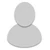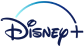## FANDOM

2,919 Pages

• Suppose I have a lua table as below:

```local data = {
{ ['name'] = "Piccaso", ['dob'] = "", ['pob'] = "Earth" };
{ ['name'] = "Optimus Prime", ['dob'] = "", ['pob'] = "Cybertron" };
{ ['name'] = "Kara Zor-El", ['dob'] = "", ['pob'] = "Krypton" };
{ ['name'] = "Pennywise", ['dob'] = "", ['pob'] = "Earth" };
{ ................... };
}
```

Now I want to create a function, which will list all the "name" value if the "pob" value is "Earth" (for example).

Sample function:

```function p.load(data)
for i, v in ipairs(data) do
if data[i]['pob'] == "Earth" then
end
end
end
return p
```

The function should iterate through the tables, and read the value of "pob", if "pob" = "Earth", but the result on the page is empty... HELP!

• There's a script error on the line with `if` statement. Any idea why?

1. ur data[i] is already in the v.
2. idk what is in ur source data, but if it is not smth like data={[somekey]={['pob']='stuff'}} then u'll get error.
3. put `local answer = ""` out of the loop or put return in the if statement.

also, click on the error message on the page to see error description and backtrace. aslo also, link would be better than piece of probably irrelevant code.

• Link to Module + Sandbox

• If I remove data[i] or data[v] and put just v, i get a different variable related script error.
• My source data is exactly the one I've shown here; but if 'somekey' is needed I can input it, a random ID number maybe!
• I probably got it!

```local p = {}

local data = {
{ ['name'] = "Piccaso", ['dob'] = "", ['pob'] = "Earth" },
{ ['name'] = "Optimus Prime", ['dob'] = "", ['pob'] = "Cybertron" },
{ ['name'] = "Kara Zor-El", ['dob'] = "", ['pob'] = "Krypton" },
{ ['name'] = "Pennywise", ['dob'] = "", ['pob'] = "Earth" },
}

for i,v in ipairs(data) do
if data[i]['pob'] == "Earth" then
end
end
end
return p
```
• Thanks a lot!

•Give Kudos to this message
You've given this message Kudos!
See who gave Kudos to this message
Community content is available under CC-BY-SA unless otherwise noted.